The Prisms ClipArt gallery includes 166 examples of polyhedrons with two parallel faces, known as the bases, and with remaining faces that are parallelograms. Prisms are named by the shape of the base.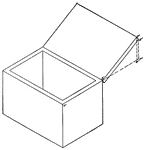### Isometric of a Box With a Cover

Isometric of a box with a cover.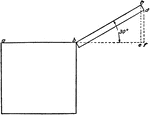### Isometric of a Box With a Cover

Isometric of a box with a cover - end view.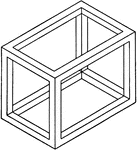### Isometric Of A Box

Illustration of the isometric of a skeleton of a box.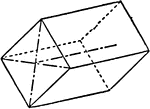### Brachy-prism and Macro-pinacoid

"This consists of four faces perpendicular to the vertical axis." -The Encyclopedia Britannica 1910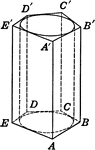### Cylinder Inscribed in Pentagonal Prism

Right circular cylinder inscribed in a pentagonal prism. Or, Pentagonal prism circumscribed about a…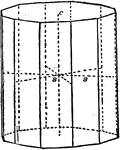### Dihexagonal Prism

Principal forms of the hexagonal system: dihexagonal prism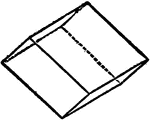### Direct Rhombohedra

"...consisting of six rhomb-shaped faces with the edges all of the equal lengths: the faces are perpendicular…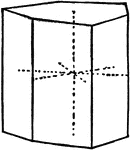### Ditrigonal Prism

Principal forms of the hexagonal system: ditrigonal prism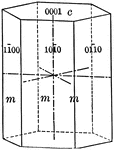### Prism of the first order

"This is a form consisting of six rectangular vertical faces each of which intersects two of the horizontal…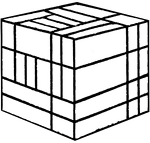### Froebel's Divided Cube (Complex)

This image shows one of Friedrich Froebel's divided cube (this one divided into many smaller cubes and…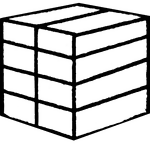### Froebel's Divided Cube (Eight Smaller Parallelograms)

This image shows one of Friedrich Froebel's divided cube (this one divided into eight smaller parallelograms).…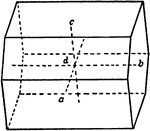### Hemi-macro Dome

Principal forms of the triclinic system: hemi-macro dome.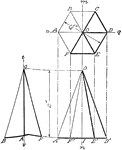### Projection Of Hexagonal Prism

Illustration of the projection of a hexagonal prism.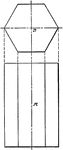### Projection of Hexagonal Bar

Projection of a hexagonal bar.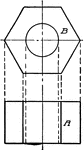### Projection of Hexagonal Nut

Projection of a hexagonal nut.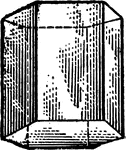### Hexagonal Prism

"Science has succeeded in classifying the thousands of known crystals in six systems, to each of which…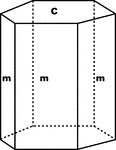### Hexagonal Prism and Basal Pinacoid

"...shows a combination of a hexagonal prism (m) with the basal pinacoid (c)." -The Encyclopedia Britannica…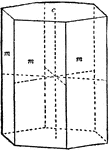### Hexagonal Prism First Order

Principal forms of the hexagonal system: hexagonal prism### Hexagonal Prism of the First Order

"Hexagonal prism of the first order, consisting of six faces also parallel to the hexad axis, but perpendicular…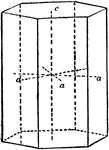### Hexagonal Prism Second Order

Principal forms of the hexagonal system: hexagonal prism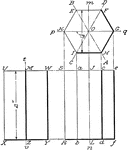### Projection Of Hexagonal Prism

Illustration of the projection of a hexagonal prism.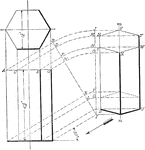### Projection Of Hexagonal Prism

Illustration of the projection of a hexagonal prism having two of its parallel sides parallel to the…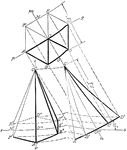### Projection Of Hexagonal Prism

Illustration of the projection of a hexagonal prism whose axis is parallel to the plane of the paper…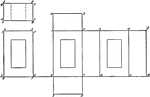### Development of Hollow Rectangular Prism

Pattern that can be used to make a hollow rectangular prism. Development of hollow rectangular prism.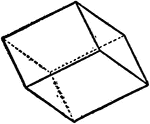### Indirect Rhombohedra

"...consisting of six rhomb-shaped faces with the edges all of the equal lengths: the faces are perpendicular…### Macro-pinacoids

Principal forms of the triclinic system: macro-pinacoids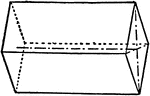### Macro-prism and Brachy-pinacoid

"This consists of four faces parallel to the brachy axis." -The Encyclopedia Britannica 1910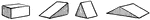### Oblique Prisms

Showing different types of oblique prisms: rectangular, right triangular, acute triangular, and obtuse…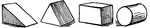### Oblique Prisms

Types of oblique prisms: right triangular prism, isosceles triangular prism, rectangular prism, cylinder.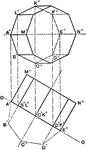### Octagonal Prism Orthagonal Projection

Three dimensional to two dimensional representation of an octagonal prism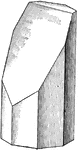### Plane Intersecting Octagonal Prism

Illustration showing the intersection of a plane with an octagonal prism.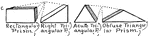### Parallel Prisms

Showing different types of parallel prisms: rectangular, right triangular, acute triangular, and obtuse…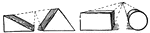### Parallel Prisms

Types of parallel prisms: right triangular prism, isosceles triangular prism, rectangular prism, cylinder.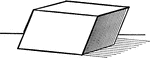### Parallelopiped

Illustration of a parallelopiped - a prism with a parallelogram as its base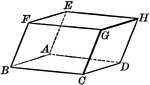### Parallelopiped

Illustration of a parallelopiped - a prism with a parallelogram as its base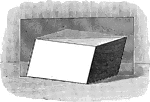### Parallelopiped with Plane Passing Through

Illustration of a parallelopiped with a plane passing through two diagonally opposite edges. The plane…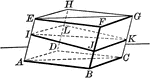### Parallelopiped with Plane Passing Through

Illustration of a parallelopiped with a plane passing through two diagonally opposite edges. The plane…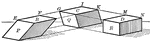### Parallelopiped Showing Volume

Illustration of a parallelopiped (a prism with a parallelogram as its base) used to demonstrate that…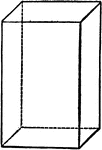### Parallelopipedon

"A parallelopipedon is a prism whose bases (ends) are parallelograms." —Hallock 1905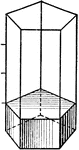### Volume Of Pentagonal Prism

Illustration used to show finding the volume of a pentagonal prism.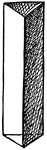### Prism

A transparent body, with usually three sides and two equal and parallel triangular ends or bases.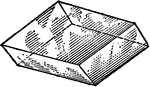### Prism

"Doubly oblique prism." — Hallock, 1905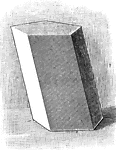### Prism

Illustration of a prism - a polyhedron of which two faces are equal polygons in parallel planes, and…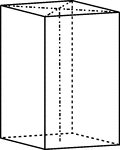### Prism and Basal Pinacoid

Holohedral orthorhombic combination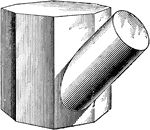### Intersecting Prism and Cylinder

Illustration of the intersection of an octagonal prism and a cylinder. The figures intersect at an oblique…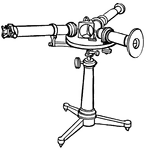### Prism Spectroscope from the Late 19th Century

"...a tube with a slit at the further end through which the light enters, and at the other end a collimating…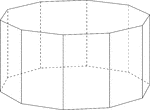### Decagonal Prism

Illustration of a decagonal prism with regular decagons for bases and rectangular faces. The hidden…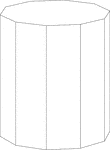### Decagonal Prism

Illustration of a right decagonal prism with regular decagons for bases and rectangular faces.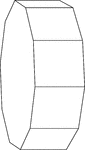### Decagonal Prism Resting On Its Side

Illustration of a right decagonal prism with regular decagons for bases and rectangular faces. The prism…### Swimming Pool Shaped Like A Decagonal Prism

Illustration of a swimming pool and water hose that is in the shape of a hollow regular decagonal prism…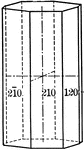### Ditetragonal prism

"The dietetragonal prism is a form consisting of eight rectangular vertical faces, each of which intersects…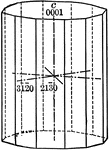### Dihexagonal prism

"The dihexagonal prism has twelve rectangular vertical faces, each of which intersects all three of…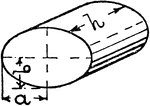### Elliptical Prism

An illustration of an elliptical prism.### First order prism

"The prism of the first order consists of four rectangular vertical faces, each of which intersects…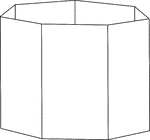### Heptagonal/Septagonal Prism

Illustration of a hollow right heptagonal/septagonal prism with regular heptagons/septagons for bases…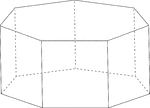### Heptagonal/Septagonal Prism

Illustration of a regular right heptagonal/septagonal prism with regular heptagons/septagons for bases…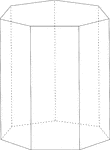### Heptagonal/Septagonal Prism

Illustration of a right heptagonal/septagonal prism with regular heptagons/septagons for bases and rectangular…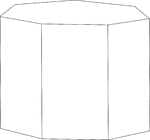### Heptagonal/Septagonal Prism

Illustration of a right heptagonal/septagonal prism with regular heptagons/septagons for bases and rectangular…